# Compress Machine Learning Model for Memory-Limited Hardware

This example shows how to reduce the size of a machine learning model for deployment to memory-limited hardware. To demonstrate the model compression workflow, the example builds models for the acoustic scene classification (ASC) task, which classifies environments from the sounds they produce. ASC is a generic multiclass classification problem that is foundational for context awareness in devices, robots, and other applications .

Assume that you want to build a model for hearing aids where the available memory size is 30 KB. First, simplify the multiclass ASC task to a binary classification problem, and them perform these steps:

• Reduce the number of features by selecting important features.

• Optimize hyperparameters with coupled constraints, which limit the size of a machine learning model.

• Quantize model parameters.

For more details on optimizing hyperparameters to reduce the memory size, see More About.

Load the `acousticscenes` data set, and display the variables in the data set.

```load("acousticscenes.mat") whos```
``` Name Size Bytes Class Attributes xEval 300x286 686400 double xTest 300x286 686400 double xTrain 1500x286 3432000 double yEval 300x1 2102 categorical yTest 300x1 2102 categorical yTrain 1500x1 3302 categorical ```

`xTrain`, `xEval`, and `xTest` contain features extracted from the TUT acoustic scene data set using wavelet scattering. `yTrain`, `yEval`, and `yTest` contain acoustic scene labels of 15 different types for `xTrain`, `xEval`, and `xTest`, respectively. In this example, you use `xTrain` and `yTrain` to train models and `xTest` and `yTest` to test the accuracy of the trained models. During the optimization step, you use `xEval` and `yEval` as a holdout validation set.

The TUT acoustic scene data set provides development data (`TUT-acoustic-scenes-2017-development` ) and test data (`TUT-acoustic-scenes-2017-evaluation` ). The development data provides a 4-fold cross-validation setup. `xTrain` and `xEval` are from the subsets of the training and evaluation sets (respectively) defined by the first fold of the cross-validation setup, and `xTest` is from the subset of the test data set. The example Acoustic Scene Recognition Using Late Fusion (Audio Toolbox) describes how you can obtain these variables from a subset of the TUT acoustic scene data set.

Normalize the data sets.

```[xTrain,mu,sigma] = normalize(xTrain); xEval = normalize(xEval,center=mu,scale=sigma); xTest = normalize(xTest,center=mu,scale=sigma);```

### Select Classification Model Types

Select types of classification models for this example by using the Classification Learner app.

1. On the Apps tab, open the apps gallery. Then, in the Machine Learning and Deep Learning group, click Classification Learner.

2. On the Classification Learner tab, in the File section, click New Session and select From Workspace. In the dialog box, specify `yTrain` as the response variable, and specify the variables in `xTrain` as predictors.

3. In the Models section of the app, click All. This option trains all the model presets available for your data set.

4. In the Train section, click Train All and select Train All.

You can compare trained models based on accuracy scores, visualize results by plotting class predictions, and check performance using the confusion matrix and ROC curve. For more details on Classification Learner, see Train Classification Models in Classification Learner App.

In this example, you work with these five model types:

• Bilayered neural network

• Linear discriminant

• Random subspace ensemble with discriminant analysis learners

• Linear SVM

• Logistic regression

Create a variable containing the model names.

```MdlNames = ["Bilayered NN","Linear Discriminant", ... "Subspace Discriminant","Linear SVM","Logistic Regression"]';```

### Train Multiclass Classification Models

Train the five models using fitting functions at the command line, and then reduce the size of the trained models by using the `compact` function. The `compact` function discards information that is not necessary for prediction.

SVM and logistic regression models support only binary classification. Therefore, use the `fitcecoc` function to train a multiclass classification model with linear SVM learners and a multiclass classification model with logistic regression learners. For the logistic regression model, use a `templateLinear` learner; in this case, you do not use the `compact` function because `fitcecoc` returns a compact model object (`CompactClassificationECOC`).

```rng("default") % For reproducibility multiMdls = cell(5,1); % Bilayered NN multiMdls{1} = compact(fitcnet(xTrain,yTrain,LayerSizes=[10 10])); % Linear Discriminant multiMdls{2} = compact(fitcdiscr(xTrain,yTrain)); % Subspace Discriminant multiMdls{3} = compact(fitcensemble(xTrain,yTrain, ... Method="Subspace",Learners="discriminant", ... NumLearningCycles=30,NPredToSample=25)); % Linear SVM multiMdls{4} = compact(fitcecoc(xTrain,yTrain)); % Logistic Regression tLinear = templateLinear(Learner="logistic"); multiMdls{5} = fitcecoc(xTrain,yTrain,Learners=tLinear);```

Specify the output display format as `bank` to display two digits after the decimal point.

`format("bank")`

Test the models with the test data set using the helper function `helperMdlMetrics`.This function returns a table of model metrics including the model accuracy as a percentage and the model size in KB. The code for the `helperMdlMetrics` function appears at the end of this example.

```multiMdlTbl = helperMdlMetrics(multiMdls,xTest,yTest); tbl1 = multiMdlTbl; tbl1.Properties.RowNames = MdlNames; disp(tbl1)```
``` Accuracy Model Size ________ __________ Bilayered NN 54.33 36.17 Linear Discriminant 53.33 2776.71 Subspace Discriminant 50.67 881.54 Linear SVM 34.33 901.90 Logistic Regression 50.00 1937.67 ```

The size of each model is more than 30 KB, and the accuracy value is approximately 50% for most models.

### Simplify Problem as Binary Classification

For the hearing aid application, assume you only want to distinguish background sounds and sounds from specific sources, instead of classifying sounds into the 15 types included in the data set. Group the types of sounds into two types (`AllAround` and `Directional`) by using the `mergecats` function.

```AllAround = ["beach","forest_path","park","office","home", ... "library","city_center","residential_area"]; Directional = ["train","bus","car","tram","grocery_store", ... "metro_station","cafe/restaurant"]; yTrainMapped = mergecats(yTrain,AllAround,"AllAround"); yTrainMapped = mergecats(yTrainMapped,Directional,"Directional"); yEvalMapped = mergecats(yEval,AllAround,"AllAround"); yEvalMapped = mergecats(yEvalMapped,Directional,"Directional"); yTestMapped = mergecats(yTest,AllAround,"AllAround"); yTestMapped = mergecats(yTestMapped,Directional,"Directional");```

Create a grouped scatter plot of the first two principal components to see whether the binary grouping works.

```figure [~,score] = pca(xTrain); gscatter(score(:,1),score(:,2),yTrainMapped) xlabel("First principal component") ylabel("Second principal component")```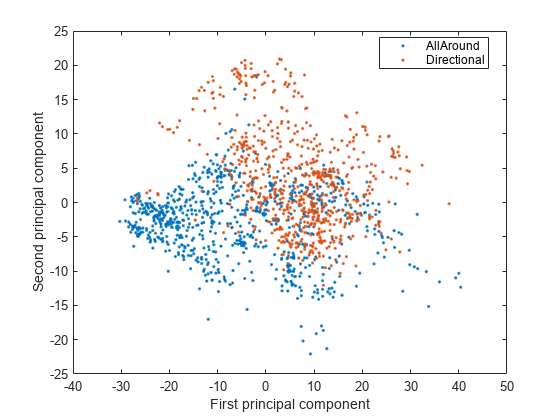### Train Binary Classification Models

Train the models for the binary sound labels `yTrainMapped`. For the linear SVM model, reduce the memory size by discarding the support vectors by using the `discardSupportVectors` function. The model can still predict new data using the linear predictor coefficients stored in the model property `Beta`. For the logistic regression model, the `fitclinear` function returns a compact model that does not store the training data.

```rng("default") binaryMdls = cell(5,1); % Bilayered NN binaryMdls{1} = compact(fitcnet(xTrain,yTrainMapped,LayerSizes=[10 10])); % Linear Discriminant binaryMdls{2} = compact(fitcdiscr(xTrain,yTrainMapped)); % Subspace Discriminant binaryMdls{3} = compact(fitcensemble(xTrain,yTrainMapped, ... Method="Subspace",Learners="discriminant",NumLearningCycles=30,NPredToSample=25)); % Linear SVM binaryMdls{4} = discardSupportVectors(compact(fitcsvm(xTrain,yTrainMapped))); % Logistic Regression binaryMdls{5} = fitclinear(xTrain,yTrainMapped,Learner="logistic");```

Test the binary classification models with the test data set `yTestMapped`.

```binaryMdlTbl = helperMdlMetrics(binaryMdls,xTest,yTestMapped); tbl2 = table(multiMdlTbl,binaryMdlTbl); tbl2.Properties.RowNames = MdlNames; tbl2.Properties.VariableNames = ["Multiclass","Binary"]; disp(tbl2)```
``` Multiclass Binary Accuracy Model Size Accuracy Model Size ______________________ ______________________ Bilayered NN 54.33 36.17 99.33 31.89 Linear Discriminant 53.33 2776.71 98.00 1314.90 Subspace Discriminant 50.67 881.54 99.33 552.08 Linear SVM 34.33 901.90 97.00 8.74 Logistic Regression 50.00 1937.67 98.67 18.60 ```

The trained models accurately classify the acoustic scenes for the binary classification problem. The linear SVM and logistic regression models are smaller than 30 KB.

### Train Models with Fewer Features

You can make machine learning models smaller without losing too much accuracy by building models using only important features. `xTrain`, `xTest`, and `xEval` include 286 features. Select 50 features by using the `fscmrmr` function.

```idx = fscmrmr(xTrain,yTrainMapped); xTrainSelected = xTrain(:,idx(1:50)); xEvalSelected = xEval(:,idx(1:50)); xTestSelected = xTest(:,idx(1:50));```

Train binary classification models using the selected features.

```rng("default") feat50binaryMdls = cell(5,1); % Bilayered NN feat50binaryMdls{1} = compact(fitcnet(xTrainSelected,yTrainMapped,LayerSizes=[10 10])); % Linear Discriminant feat50binaryMdls{2} = compact(fitcdiscr(xTrainSelected,yTrainMapped)); % Subspace Discriminant feat50binaryMdls{3} = compact(fitcensemble(xTrainSelected,yTrainMapped, ... Method="Subspace",Learners="discriminant",NumLearningCycles=30,NPredToSample=25)); % Linear SVM feat50binaryMdls{4} = discardSupportVectors(compact(fitcsvm(xTrainSelected,yTrainMapped))); % Logistic Regression feat50binaryMdls{5} = fitclinear(xTrainSelected,yTrainMapped,Learner="logistic");```

Test the models with the test data set `yTestMapped`.

```feat50binaryMdlTbl = helperMdlMetrics(feat50binaryMdls,xTestSelected,yTestMapped); tbl3 = table(multiMdlTbl,binaryMdlTbl,feat50binaryMdlTbl); tbl3.Properties.RowNames = MdlNames; tbl3.Properties.VariableNames = ["Multiclass","Binary","50 Features"]; disp(tbl3)```
``` Multiclass Binary 50 Features Accuracy Model Size Accuracy Model Size Accuracy Model Size ______________________ ______________________ ______________________ Bilayered NN 54.33 36.17 99.33 31.89 90.67 11.38 Linear Discriminant 53.33 2776.71 98.00 1314.90 95.33 51.70 Subspace Discriminant 50.67 881.54 99.33 552.08 91.33 541.91 Linear SVM 34.33 901.90 97.00 8.74 96.33 4.82 Logistic Regression 50.00 1937.67 98.67 18.60 97.00 12.18 ```

In addition to the linear SVM and logistic regression models, the bilayered neural network model is also smaller than 30 KB. However, reducing the number of features causes the accuracy to decrease in the trained models.

Restore the default display format.

`format("default")`

### Optimize Neural Network with Coupled Constraints

Find optimal model hyperparameters while limiting the memory use of the models. The constraints depend on the type of machine learning model. For example, you can limit the number of support vectors for an SVM model or limit the number of parameters in a neural network model. For more details on Bayesian optimization and an example for an SVM model, see Constraints in Bayesian Optimization. This example shows constraint-coupled optimization for a bilayered neural network model.

For constraint-coupled optimization, specify the hyperparameters to optimize and define a customized objective function. Then, use the `bayesopt` function to find the optimal hyperparameters based on the objective function.

First, get the default hyperparameters of the bilayered neural network model by using the `hyperparameters` function.

`params_bilayeredNet = hyperparameters("fitcnet",xTrainSelected,yTrainMapped);`

Modify the first, third, and ninth hyperparameters, which correspond to `NumLayers`, `Standardize`, and `Layer_3_Size`, so that they are not optimized. In this way, you can build a bilayered model and use training data without standardization. The training data is already standardized.

```params_bilayeredNet(1).Range = [1 2]; % NumLayers params_bilayeredNet(1).Optimize = false; params_bilayeredNet(3).Optimize = false; % Standardize params_bilayeredNet(9).Optimize = false; % Layer_3_Size```

Use the customized objective function `helperOptimizeConstrainedBilayer`, which trains a bilayered neural network model using a given set of parameters for the training data set, and returns the loss for the holdout validation set. The code for the `helperOptimizeConstrainedBilayer` function appears at the end of this example. The function also accepts the upper limit for the number of weight parameters in the model and returns a constraint value. A positive constraint value indicates that the number of parameters is greater than the specified limit.

Define a function handle `fun` that takes the hyperparameters and calls the `helperOptimizeConstrainedBilayer` function. Specify the upper limit for the number of weight parameters as 300.

`fun = @(params)helperOptimizeConstrainedBilayer(params,xTrainSelected,yTrainMapped,xEvalSelected,yEvalMapped,300);`

When you call the `bayesopt` function, specify the objective function as `fun` and specify the hyperparameters as `params_bilayeredNet`. Also, specify `NumCoupledConstraints` as 1 to indicate that the objective function has one coupled constraint. For reproducibility, set the random seed and use the `expected-improvement-plus` acquisition function.

```rng("default") resultNN = bayesopt(fun,params_bilayeredNet, ... AcquisitionFunctionName="expected-improvement-plus", ... NumCoupledConstraints=1);```
```|==================================================================================================================================================| | Iter | Eval | Objective | Objective | BestSoFar | BestSoFar | Constraint1 | Activations | Lambda | Layer_1_Size | Layer_2_Size | | | result | | runtime | (observed) | (estim.) | | | | | | |==================================================================================================================================================| | 1 | Infeas | 0.076667 | 4.1321 | NaN | 0.076667 | 2.4e+03 | none | 7.6806e-06 | 15 | 115 | | 2 | Best | 0.07 | 1.4299 | 0.07 | 0.070445 | -196 | none | 0.0001221 | 2 | 1 | | 3 | Infeas | 0.46667 | 0.086145 | 0.07 | 0.070862 | 1.39e+03 | sigmoid | 45.438 | 26 | 14 | | 4 | Best | 0.063333 | 1.6533 | 0.063333 | 0.063353 | -52.5 | tanh | 2.6069e-05 | 4 | 8 | | 5 | Accept | 0.11333 | 1.8806 | 0.063333 | 0.063423 | -58.5 | relu | 2.2423e-05 | 4 | 7 | | 6 | Accept | 0.07 | 1.4079 | 0.063333 | 0.063344 | -196 | none | 0.0001411 | 2 | 1 | | 7 | Infeas | 0.046667 | 1.7468 | 0.063333 | 0.06318 | 1.95e+04 | tanh | 1.2269e-07 | 300 | 16 | | 8 | Infeas | 0.11333 | 5.8725 | 0.063333 | 0.063575 | 9.47e+04 | tanh | 0.045218 | 298 | 267 | | 9 | Accept | 0.46667 | 0.029372 | 0.063333 | 0.063332 | -196 | none | 9.1357 | 2 | 1 | | 10 | Infeas | 0.46667 | 0.030363 | 0.063333 | 0.063332 | 1.42e+03 | relu | 3.0052 | 30 | 7 | | 11 | Best | 0.046667 | 2.4672 | 0.046667 | 0.046678 | -172 | relu | 6.691e-09 | 2 | 7 | | 12 | Accept | 0.046667 | 1.2965 | 0.046667 | 0.046675 | -52.5 | tanh | 6.7859e-09 | 4 | 8 | | 13 | Accept | 0.086667 | 3.0389 | 0.046667 | 0.046686 | -172 | relu | 1.1251e-07 | 2 | 7 | | 14 | Accept | 0.46667 | 0.03666 | 0.046667 | 0.04668 | -58.5 | tanh | 60.245 | 4 | 7 | | 15 | Best | 0.03 | 1.4417 | 0.03 | 0.030086 | -58.5 | tanh | 0.0011383 | 4 | 7 | | 16 | Infeas | 0.12333 | 0.18129 | 0.03 | 0.03007 | 296 | sigmoid | 6.766e-09 | 10 | 8 | | 17 | Accept | 0.076667 | 0.90302 | 0.03 | 0.030071 | -146 | none | 8.2973e-09 | 3 | 1 | | 18 | Best | 0.023333 | 1.2746 | 0.023333 | 0.026599 | -58.5 | tanh | 0.0009958 | 4 | 7 | | 19 | Accept | 0.026667 | 1.2009 | 0.023333 | 0.02661 | -52.5 | tanh | 0.0009402 | 4 | 8 | | 20 | Accept | 0.05 | 1.467 | 0.023333 | 0.026601 | -226 | sigmoid | 1.086e-05 | 1 | 8 | |==================================================================================================================================================| | Iter | Eval | Objective | Objective | BestSoFar | BestSoFar | Constraint1 | Activations | Lambda | Layer_1_Size | Layer_2_Size | | | result | | runtime | (observed) | (estim.) | | | | | | |==================================================================================================================================================| | 21 | Accept | 0.036667 | 1.2228 | 0.023333 | 0.027248 | -110 | tanh | 0.00090677 | 3 | 8 | | 22 | Infeas | 0.053333 | 6.5087 | 0.023333 | 0.027181 | 1.41e+04 | tanh | 0.00048938 | 283 | 1 | | 23 | Infeas | 0.12333 | 0.39799 | 0.023333 | 0.027429 | 1.71e+04 | relu | 7.1367e-09 | 238 | 23 | | 24 | Accept | 0.076667 | 1.0528 | 0.023333 | 0.029543 | -248 | none | 6.7138e-07 | 1 | 1 | | 25 | Accept | 0.046667 | 1.4113 | 0.023333 | 0.02962 | -226 | tanh | 1.1434e-07 | 1 | 8 | | 26 | Accept | 0.043333 | 1.4368 | 0.023333 | 0.029659 | -168 | sigmoid | 9.1787e-07 | 2 | 8 | | 27 | Accept | 0.043333 | 0.79319 | 0.023333 | 0.029584 | -226 | tanh | 0.0018534 | 1 | 8 | | 28 | Infeas | 0.06 | 4.1008 | 0.023333 | 0.030036 | 1.31e+04 | sigmoid | 2.3192e-06 | 257 | 2 | | 29 | Accept | 0.066667 | 1.4127 | 0.023333 | 0.026647 | -226 | tanh | 0.00050488 | 1 | 8 | | 30 | Accept | 0.036667 | 0.8016 | 0.023333 | 0.028015 | -52.5 | tanh | 0.0044111 | 4 | 8 | ```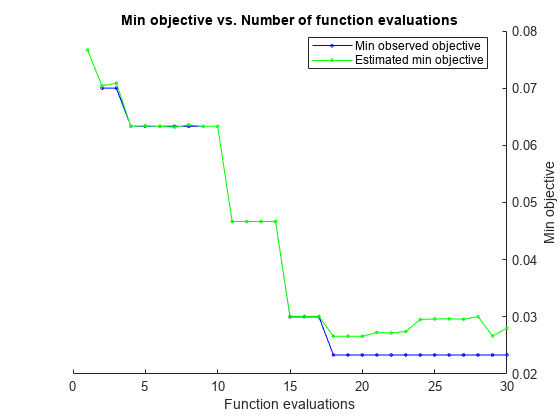```__________________________________________________________ Optimization completed. MaxObjectiveEvaluations of 30 reached. Total function evaluations: 30 Total elapsed time: 66.903 seconds Total objective function evaluation time: 50.7154 Best observed feasible point: Activations Lambda Layer_1_Size Layer_2_Size ___________ _________ ____________ ____________ tanh 0.0009958 4 7 Observed objective function value = 0.023333 Estimated objective function value = 0.029092 Function evaluation time = 1.2746 Observed constraint violations =[ -58.500000 ] Best estimated feasible point (according to models): Activations Lambda Layer_1_Size Layer_2_Size ___________ _________ ____________ ____________ tanh 0.0011383 4 7 Estimated objective function value = 0.028015 Estimated function evaluation time = 1.3037 Estimated constraint violations =[ -58.501089 ] ```

`bayesopt` finds optimal hyperparameters that minimize an error in the holdout validation set and satisfy the constraint. Extract the best point in the optimization results `resultNN` by using the `bestPoint` function.

`[optimalParams,CriterionValue1,iteration] = bestPoint(resultNN)`
```optimalParams=1×4 table Activations Lambda Layer_1_Size Layer_2_Size ___________ _________ ____________ ____________ tanh 0.0011383 4 7 ```
```CriterionValue1 = 0.0332 ```
```iteration = 15 ```

Train the bilayered neural network model with the optimal hyperparameters.

```rng("default") modelNNOpt = compact(fitcnet(xTrainSelected,yTrainMapped, ... Activations=char(optimalParams.Activations), ... LayerSizes=[optimalParams.Layer_1_Size optimalParams.Layer_2_Size], ... Lambda=optimalParams.Lambda));```

Find the accuracy and size of the trained model.

`OptimizedNNAccuracy = (1-loss(modelNNOpt,xTestSelected,yTestMapped))*100`
```OptimizedNNAccuracy = 93.3333 ```
`OptimizedNNSize = whos("modelNNOpt").bytes/1024`
```OptimizedNNSize = 8.3555 ```

### Quantize Model Parameters with Simulink Block

You can also reduce the memory footprint of a machine learning model by quantizing model parameters with a Simulink block. Statistics and Machine Learning Toolbox™ provides various prediction blocks that allows you to import a trained machine learning model into a Simulink model. In the prediction blocks, you can specify the data types for some or all model parameters as single-precision, fixed-point, half-precision, and so on. For an example of fixed-point conversion, see Human Activity Recognition Simulink Model for Fixed-Point Deployment.

This example provides the Simulink model `slexAcousticSceneClassificationNNPredictExample.slx`, which includes the ClassificationNeuralNetwork Predict block. Open this model.

```SimMdlName = 'slexAcousticSceneClassificationNNPredictExample'; open_system(SimMdlName)```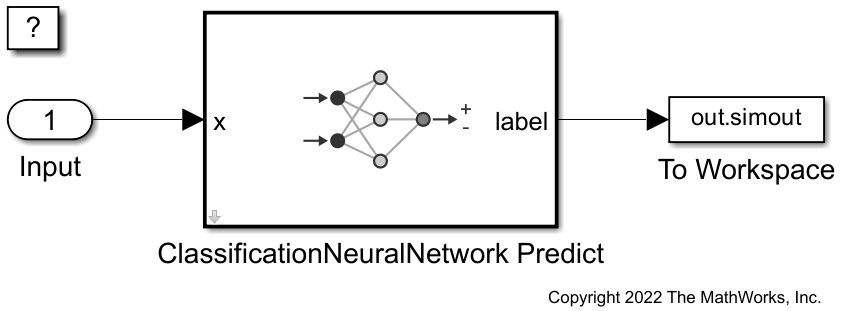Double-click the ClassificationNeuralNetwork Predict block to open the Block Parameters dialog box. You can specify the data types for the model parameters in the Data Types tab. to reduce the memory size, specify the data types for the layers as `single`. For details on specifying data types, see Specify Data Types Using Data Type Assistant (Simulink).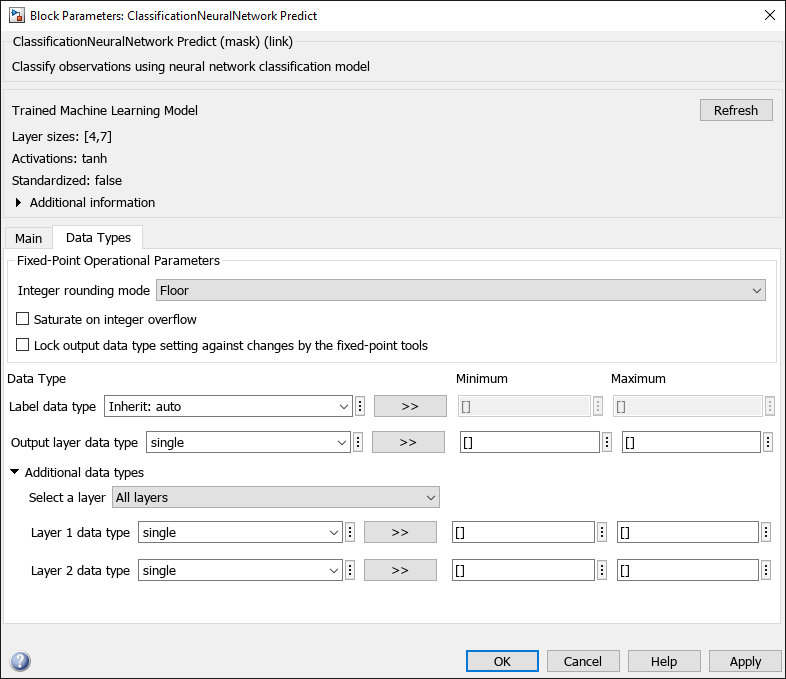Prepare the input data for the Simulink model. Convert the predictor data (`xTestSelected`) to single precision by using the `single` function.

```soundInput.time = (0:size(xTestSelected,1)-1)'; soundInput.signals(1).values = single(xTestSelected); soundInput.signals(1).dimensions = size(xTestSelected,2);```

Simulate the Simulink model and assign the result to the `out` variable.

`out = sim(SimMdlName);`

Find the accuracy of the predict block using the data logged in the To Workspace (Simulink) block.

```pred = categorical(out.simout.Data,unique(out.simout.Data),["AllAround","Directional"]); QuantizedNNAccuracy = sum(pred == yTestMapped)/length(yTestMapped)*100```
```QuantizedNNAccuracy = 93.3333 ```

Find the size of the quantized model parameters.

```p = Simulink.Mask.get("slexAcousticSceneClassificationNNPredictExample/ClassificationNeuralNetwork Predict"); vars = p.getWorkspaceVariables; blockParams = vars(end).Value; save("params.mat","blockParams") s = dir("params.mat"); QuantizedNNSize = s.bytes/1024```
```QuantizedNNSize = 2.5000 ```

### Model Compression Summary

Display the changes in model size and accuracy during the model compression workflow for the bilayered neural network model. In general, the model loses some accuracy as you apply additional model compression schemes.

```NNccuracy = [multiMdlTbl{1,"Accuracy"} binaryMdlTbl{1,"Accuracy"} ... feat50binaryMdlTbl{1,"Accuracy"} ... OptimizedNNAccuracy QuantizedNNAccuracy]; NNSize = [multiMdlTbl{1,"Model Size"} binaryMdlTbl{1,"Model Size"} ... feat50binaryMdlTbl{1,"Model Size"} ... OptimizedNNSize QuantizedNNSize]; ModelType = ["Multiclass","Binary","50 Features","Optimized","Quantized"]; figure yyaxis left b = bar(NNSize); xtips = b.XEndPoints; ytips = b.YEndPoints; labels = string(round(b.YData,2)); text(xtips,ytips,labels,HorizontalAlignment="center",VerticalAlignment="bottom", ... Color='#0072BD') ylabel("Model Size [KB]") yyaxis right plot(NNccuracy,"-o") ylabel("Accuracy [%]") xticklabels(ModelType) grid on```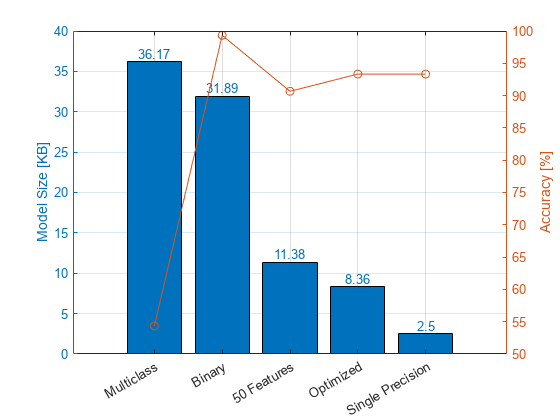For the bilayered neural network model, the model size decreases to less than 30 KB after you reduce the number of features. The constrained optimization and quantization with a Simulink block further reduce the model size.

The accuracy of the initial multiclass classification model is lower compared to the other models, because the multiclass model classifies sounds into 15 types. After you simplify the multiclass problem into a binary classification problem, the models accurately classify more than 90% of the test data. Reducing the number of features leads to a loss of model accuracy, but the constrained optimization step improves accuracy, and the quantization does not reduce accuracy.

### Helper Functions

The `helperMdlMetrics` function takes a cell array of trained models (`Mdls`) and test data sets (`X` and `Y`) and returns a table of model metrics that includes the model accuracy as a percentage and the model size in KB. The helper function uses the `whos` function to estimate the model size. However, the size returned by the `whos` function can be larger than the actual model size required in the generated code for deployment. For example, the generated code does not include information that is not needed for prediction. Consider a `CompactClassificationECOC` model that uses logistic regression learners. The binary learners in a `CompactClassificationECOC` model object in the MATLAB workspace contain the `ModelParameters` property. However, the model prepared for deployment in the generated code does not contain this property.

```function tbl = helperMdlMetrics(Mdls,X,Y) numMdl = length(Mdls); metrics = NaN(numMdl,2); for i = 1 : numMdl Mdl = Mdls{i}; MdlInfo = whos("Mdl"); metrics(i,:) = [(1-loss(Mdl,X,Y))*100 MdlInfo.bytes/1024]; end tbl = array2table(metrics, ... VariableNames=["Accuracy","Model Size"]); end```

The `helperOptimizeConstrainedBilayer` function trains a bilayered neural network model using a given set of parameters for the training data, and returns the loss for the holdout validation set. In addition, the function accepts the upper limit (`maxSize`) for the number of weight parameters in the model and returns a constraint value. A positive constraint value indicates that the number of parameters is greater than the specified limit `maxSize`.

```function [objective,constraint] = helperOptimizeConstrainedBilayer(params,xTrain,yTrain,xEval,yEval,maxSize) mdl = fitcnet(xTrain,yTrain, ... Activations=char(params.Activations), ... LayerSizes=[params.Layer_1_Size params.Layer_2_Size], ... Lambda=params.Lambda); objective = loss(mdl,xEval,yEval); numClasses = size(unique(yTrain),1); sizeEst = size(xTrain,2)*params.Layer_1_Size + ... params.Layer_1_Size*params.Layer_2_Size + ... params.Layer_2_Size*numClasses; constraint = sizeEst - maxSize - 0.5; end```

For constraint-coupled optimization, you can consider minimizing these hyperparameters to limit the memory use, depending on the type of machine learning model:

• Decision tree — Minimum number of leaf node observations (MinLeafSize) and the maximum number of decision splits (MaxNumSplits). A decision tree model has a small memory footprint.

• Linear discriminant and logistic regression — Number of features and classes. Both a linear discriminant model and a logistic regression model have a small to medium memory footprint.

• Shallow neural network — Number of fully connected layers and the number of hidden units in each layer (LayerSizes). A shallow neural network model has a small to medium memory footprint.

• k-nearest neighbor — Training data size, the number of nearest neighbors (NumNeighbors), and the maximum number of data points in the leaf node for the Kd-tree algorithm (BucketSize). A k-nearest neighbor model has a medium memory footprint.

• Support vector machine (SVM) — Number of support vectors determined by the box constrains (BoxConstraint). An SVM has a medium to large memory footprint. For an SVM model that uses the linear kernel function, you can reduce the footprint by discarding support vectors from the model using the `discardSupportVectors` function. The reduced SVM model can still predict new data using predictor coefficients (`Beta` property) stored in the model.

• Ensemble — Number of learners and the size of each learner determined by NumLearningCycles and Learners. An ensemble has a medium to large memory footprint.

• Gaussian process regression (regression only) — Size of the active set (ActiveSetSize). A Gaussian process regression model has a medium to large memory footprint.

Several factors determine the memory use of a machine learning model. However, in general, the memory footprint for a decision tree model is small. A linear discriminant model, logistic regression model, and shallow neural network model have a small to medium memory footprint, and a k-nearest neighbor model has a medium memory footprint. An SVM, ensemble, and Gaussian process model have a medium to large memory footprint. For an SVM model that uses the linear kernel function, you can discard support vectors from the model to reduce the footprint by using the `discardSupportVectors` function. The reduced SVM model can still predict new data using predictor coefficients (`Beta` property) stored in the model.

For deployment to memory-limited hardware, a recommended practice is to specify training data using a matrix, not a table. If you specify training data using a table, some model properties, such as `PredictorNames`, can take a considerable proportion of the model memory footprint.

### References

 Mesaros, Annamaria, Toni Heittola, and Tuomas Virtanen. Acoustic Scene Classification: An Overview of DCASE 2017 Challenge Entries. In proc. International Workshop on Acoustic Signal Enhancement, 2018.

 Lostanlen, Vincent, and Joakim Anden. Binaural Scene Classification with Wavelet Scattering. Technical Report, DCASE2016 Challenge, 2016.